# Increasing, Decreasing and Constant Functions

Previously you learned about functions, graph of functions. In this lesson, you will learn about some function types such as increasing functions, decreasing functions and constant functions. These concepts are explained with examples and graphs of the specific functions where ever necessary.

## Increasing, Decreasing and Constant Functions

Functions are increasing, decreasing and constant when you plot the graph of the function in a coordinate system. Let’s define the meaning of these functions.

Increasing function

A function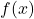is increasing in an interval for any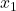and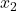• if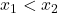• implies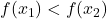Example:

Let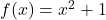be a function. Find all the values for the function to plot the graph.

The graph of the function will look like the following.

In the above graph, the function is increasing between the interval of (0, 2).

The value ofis 0 andis 3,

The value of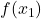is 1 and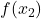is 5.

Therefore,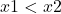impliesis true and it is an increasing function.

Decreasing Function

A functionis decreasing in an interval for anyand• if• implies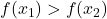Example:

Consider a function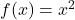. The function is a parabola. Let’s draw the graph of this function in a Cartesian plane or co-ordinate system.

Before plotting the graph, you need to find points for the graph of the function. A table of points is given below.

The graph of the parabola is given below.

In the above graph, the function is decreasing between the interval ( -2, 0).

The value ofis -2 and the value ofis 0.

The value ofis 4 and the value ofis 0.

Thenis true andis also true. Hence, the function is a decreasing function between the interval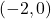.

Constant Function

The function is a constant function in an interval for someand• if• implies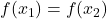This is simplest form of graph of a function and such a function is always a straight line on the coordinate system.

Let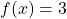be the constant function. It means for any value of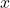in the domain, the value ofis 3.

The graph of constant function is given below.

In the above graph of the constant function.

The value ofis 1 and the value ofis 2.

The value ofis 3 and the value ofis also 3.

Therefore,andimplies that the function is a constant function.